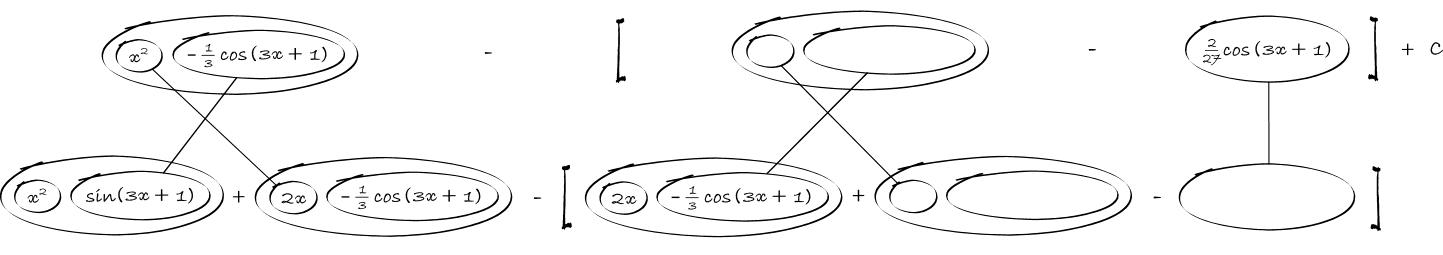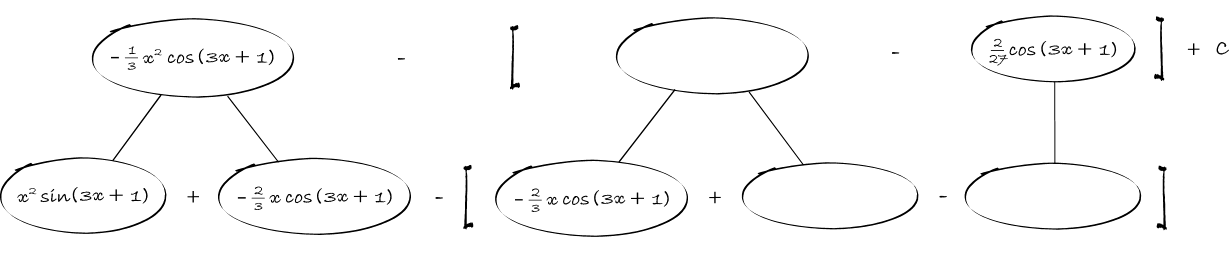# Thread: Integration by parts

1. ## Integration by parts

Can someone help me with this integration by parts equation x^2sin(3x+1) Thank you.

2.Originally Posted by Link88Can someone help me with this integration by parts equation x^2sin(3x+1) Thank you.
Let $\displaystyle x^2=u\implies{2xdx=du}$ and $\displaystyle dv=\sin(3x+1)dx\implies{v=\frac{-1}{3}\cos(3x+1)}$
Can you go from there? You will need to do it twice.

3. Thats the part I get stuck at is doing it twice it gets confusing

4.Originally Posted by Mathstud28Let $\displaystyle x^2=u\implies{2xdx=du}$ and $\displaystyle dv=\sin(3x+1)dx\implies{v=\frac{-1}{3}\cos(3x+1)}$
Can you go from there? You will need to do it twice.
Can you show me the second part thats where i got stuck at. Thank you.

5.Originally Posted by Link88Can you show me the second part thats where i got stuck at. Thank you.
You need to use integration by parts twice, applying once using Mathstud28 choice of u and v gives:

$\displaystyle I=\int x^2 \sin(3x+1) \ dx=-x^2 \left[ \frac{1}{3} \cos(3x+1)\right] + \int 2x \left[ \frac{1}{3} \cos(3x+1)\right] \ dx$

Use integration by parts again to do the integral on the right.

CB

6. Don't integrate - balloontegrate!Straight lines integrate up, differentiate down (making it easier to check). The triangular networks satisfy the product rule - you may prefer to draw a zoomed-out version of these...The challenge of integration by parts is simply to fill out a product rule shape starting with one of the bottom balloons instead of (as happens in differentiation) the top one.

See Balloon Calculus: worked examples from past papers

or http://www.mathhelpforum.com/math-he...tegration.html

to see the process.

7. Have you ever seen tabular integration. Here it is for fun.

Let $\displaystyle u=x^{2}, \;\ dv=v'dx=sin(3x+1)$

Take the derivatives of u until you get to 0. Take the antiderivatives of v'.

$\displaystyle \text{sign \;\ \;\ u and its derivatives \;\ \;\ \;\ v' and its antiderivatives }$

$\displaystyle \begin{array}{ccc}+& \;\ \;\ \;\ x^{2}\searrow& \;\ \;\ sin(3x+1)\\-&2x\searrow&\frac{-1}{3}cos(3x+1)\\-&2\searrow&\frac{-1}{9}sin(3x+1)\\-&0&\frac{1}{27}cos(3x+1)\end{array}$

Now, take the signed products and add up the diagonal entries:

$\displaystyle \frac{-1}{3}x^{2}cos(3x+1)+\frac{2}{9}xsin(3x+1)+\frac{2} {27}cos(3x+1)+C$

#### Search Tags

integration, parts